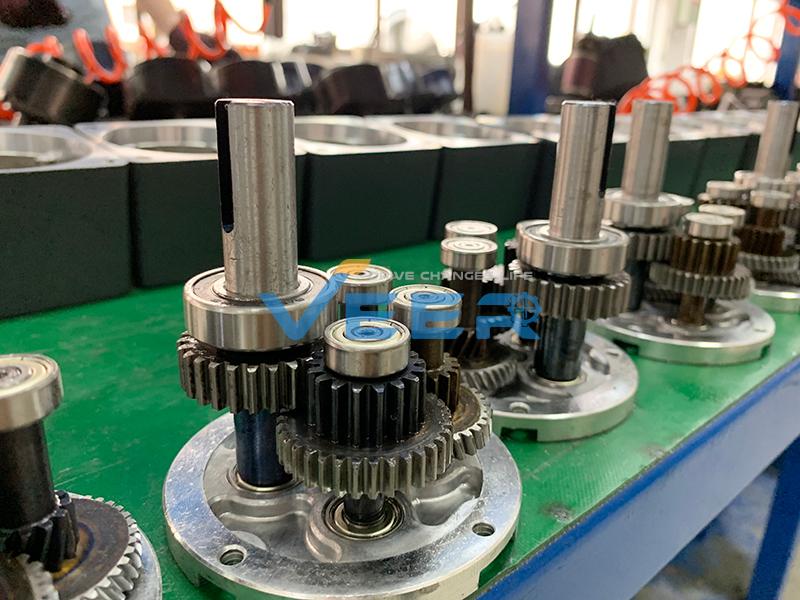How to calculate gear ratio of gear reduction motor?

The “reduction” or gear ratio is calculated by dividing the number of teeth on the large gear by the number of teeth on the small gear. For example, if an electric motor drives a 13-tooth pinion gear that meshes with a 65-tooth gear, a reduction of 5:1 is achieved (65 / 13 = 5). If the electric motor speed is 1,500 rpm, the gearbox reduces this speed by five times to 300 rpm. If the motor torque is 10 lb-in, the gearbox increases this torque by a factor of five to 50 lb-in (before subtracting out gearbox efficiency losses).How to adjust gear motor rotate speed? We are gear motor supplier.